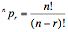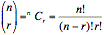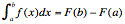Te Kete Ipurangi
Communities
Schools

### Te Kete Ipurangi user options:

Activities:

Activities:

Maths activities:

Stats activities:

# Level 8 Achievement objectives

M8-1 |  M8-2 |  M8-3 |  M8-4 |  M8-5 |  M8-6 |  M8-7 |  M8-8 |  M8-9 |

## Achievement objective M8-1

In a range of meaningful contexts, students will be engaged in thinking mathematically and statistically. They will solve problems and model situations that require them to:

• apply the geometry of conic sections.

### Indicators

• Demonstrates understanding of conic sections, including circles, ellipses, parabolas, hyperbolas, including links to transformations and definitions of loci.
• Connects equations in parametric and Cartesian form with the graphs of conic sections, including understanding the standard equation of each type of conic section.
• Identifies and uses key features of conic sections, such as vertices, foci, asymptotes, intercepts and eccentricity.

## Achievement objective M8-2

In a range of meaningful contexts, students will be engaged in thinking mathematically and statistically. They will solve problems and model situations that require them to:

• display and interpret the graphs of functions with the graphs of their inverse and/or reciprocal functions.

### Indicators

• Demonstrates understanding of inverse and/or reciprocal functions, including those of:
• polynomial
• exponential y = eax or y = bx
• logarithmic
• rectangular hyperbola• trigonometric equations of the form y = Asin(Bx ) + C for sin, cos and tan functions with x in radians or degrees
• f(x) = √ x
• Makes graphical and algebraic connections between a function and its inverse, including the consideration of restrictions on the domain of a function so that its inverse is also a function.

## Achievement objective M8-3

In a range of meaningful contexts, students will be engaged in thinking mathematically and statistically. They will solve problems and model situations that require them to:

• use permutations and combinations.

### Indicators

• Understands the basic terms and notation of permutations and combinations, including:,• Selects and uses appropriate methods to find numbers of distinct arrangements or selections from a given number of objects in order to solve problems and calculate probabilities, including:
• the multiplication principle
• factorials
• permutations, including fixed position of items, groups of items, and identical items
• combinations including restrictions on the selections and selecting from more than one group.

## Achievement objective M8-4

In a range of meaningful contexts, students will be engaged in thinking mathematically and statistically. They will solve problems and model situations that require them to:

• use curve fitting, log modelling, and linear programming techniques.

### Indicators

• Solves problems using curve fitting and log modelling.
• Solves problems using linear programming techniques:
• determining the variables of interest
• translating word problems into mathematical expressions or equations
• manipulating linear inequalities
• graphically representing linear systems in two variables that include inequalities
• solving simultaneous linear, and non-linear equations
• identifying feasible regions
• optimising a function subject to the constraints of a system
• interpreting solutions of linear systems in context, including situations with multiple solutions.

## Achievement objective M8-5

In a range of meaningful contexts, students will be engaged in thinking mathematically and statistically. They will solve problems and model situations that require them to:

• develop network diagrams to find optimal solutions, including critical paths.

### Indicators

• Uses network diagrams to find optimal solutions, including critical paths.
• Uses critical path analysis to schedule the tasks in a project to meet particular constraints.
• Constructs network diagrams for critical path analysis from precedence tables.
• Uses critical path analysis to establish the tasks on the critical path, the critical events.
• Uses critical path analysis to find the float times for activities that are not on the critical path.

## Achievement objective M8-6

In a range of meaningful contexts, students will be engaged in thinking mathematically and statistically. They will solve problems and model situations that require them to:

• manipulate trigonometric expressions.

### Indicators

• Solves problems that involve manipulating trig expressions using a variety of trig identities, including:
• the Pythagorean identities
• compound angle formula
• double angle formula
• sums and products
• reciprocal trigonometric functions.
• Identifies and uses appropriate key features of trigonometric functions, that is, amplitude, period and frequency.

## Achievement objective M8-7

In a range of meaningful contexts, students will be engaged in thinking mathematically and statistically. They will solve problems and model situations that require them to:

• form and use trigonometric, polynomial, and other non-linear equations.

### Indicators

• Solves contextual problems by forming and solving equations.
• Solves quadratic and cubic equations with complex roots. These equations are likely to involve real coefficients so that solutions are complex conjugate pairs.
• Analyses the existence of solutions in the context of the situation.
• Non-linear equations include:
• exponential
• logarithmic
• rational
• surd
• hyperbolic.

## Achievement objective M8-8

In a range of meaningful contexts, students will be engaged in thinking mathematically and statistically. They will solve problems and model situations that require them to:

• form and use systems of simultaneous equations, including three linear equations and three variables, and interpret the solutions in context.

### Indicators

• Translates contextual problems into systems of linear equations.
• Solves problems that can be modelled by systems of three linear equations with up to three unknowns.
• Chooses appropriate methods and uses them to solve simultaneous equations.
• Understands the process of solving systems of equations algebraically.
• Manipulates equations.
• Interprets solution(s) of a linear system in context.
• Identifies solutions that may be unique, infinite or non-existent.
• Explains the nature of solution(s) including consistency and uniqueness and interprets this in context, including 3D.

## Achievement objective M8-9

In a range of meaningful contexts, students will be engaged in thinking mathematically and statistically. They will solve problems and model situations that require them to:

• manipulate complex numbers and present them graphically.

### Indicators

• Uses and manipulates surds and other irrational numbers.
• Interprets and uses graphical representations of complex numbers, using polar and rectangular form on an Argand diagram.
• Uses and manipulates complex numbers in rectangular form and polar form and makes links with their graphical representation.
• Finds roots over the complex number system for polynomial equations with real coefficients, making links with their graphs.

## Achievement objective M8-10

In a range of meaningful contexts, students will be engaged in thinking mathematically and statistically. They will solve problems and model situations that require them to:

• identify discontinuities and limits of functions.

### Indicators

• Links features of graphs with the limiting behaviour of functions.
• Uses limiting features of functions to sketch graphs.
• Finds limits algebraically, graphically, and numerically by considering behaviour as:
• x approaches a specific value from above and below
• x tends towards +∞ or -∞
• Demonstrates understanding of continuity at a point (the limit as x tends to a of f(x) = f(a)).
• Identifies discontinuities graphically or algebraically.
• Informally links concepts of continuity and differentiability  M7-9.
• Determines the continuity and differentiability of a function.

## Achievement objective M8-11

In a range of meaningful contexts, students will be engaged in thinking mathematically and statistically. They will solve problems and model situations that require them to:

• choose and apply a variety of differentiation, integration, and anti-differentiation techniques to functions and relations, using both analytical and numerical methods.

### Indicators

• Understands and uses a range of differentiation techniques including:
• first principles (only for polynomials of degree ≤ 3)
• product rule, quotient rule, chain rule
• other techniques including implicit or parametric differentiation, for example, conic relations.
Functions include:
• polynomials
• rational
•  ex
•  ln(x)
• trig functions
•  xn, n ∈ R .
• Develops an understanding of integration by:
• using numerical methods for finding areas under curves (rectangle rule, trapezium rule, and Simpson’s rule)
• relating the definite integral to the limit of a sum (Riemann Integral)
• linking integration and anti-differentiation using the fundamental theorem of calculus, including:
• Part 1: If f is continuous on [a, b] and F(x) =for [a, b] then F'(x) = f(x) (explores using technology) (Note: this guarantees that an anti-derivative does exist for Part 2.)
• Part 2: If F' = f then•  Uses a variety of integration techniques for functions including:
• polynomials
• xn, n ∈ R
• ex
• sin(x)
• cos(x)
• f ' ( g ( x ) ) ( g ' ( x ) )
• f'(x)/f(x)
Applications include:
• finding areas under and between curves
• (related) rates of change
• kinematics.

## Achievement objective M8-12

In a range of meaningful contexts, students will be engaged in thinking mathematically and statistically. They will solve problems and model situations that require them to:

• form differential equations and interpret the solutions.

### Indicators

• Uses knowledge of anti-differentiation to form and solve differential equations of the type:
• with the rate of change directly or inversely proportional to the variable of interest
• first and second order with variables easily separated for functions including those listed in  M8-11.
• Applies boundary or initial conditions to solutions of differential equations.
• Distinguishes between families of solutions and exact solutions using given boundary or initial conditions and interprets these solutions.
• Links families of solutions to the gradient field of the differential equation.

## Achievement objective S8-1

In a range of meaningful contexts, students will be engaged in thinking mathematically and statistically. They will solve problems and model situations that require them to:

• Carry out investigations of phenomena, using the statistical enquiry cycle:
• A. conducting experiments using experimental design principles, conducting surveys, and using existing data sets
• B. finding, using, and assessing appropriate models (including linear regression for bivariate data and additive models for time-series data), seeking explanations and making predictions
• C. using informed contextual knowledge, exploratory data analysis, and statistical inference
• D. communicating findings and evaluating all stages of the cycle.

### Indicators

• Uses the statistical enquiry cycle.
• Conducts experiments using experimental design principles to find solutions to problems:
• Poses investigative questions using the statistical enquiry cycle.
• Defines and identifies variables that need to be accounted for in the experiment.
• Designs experiments, taking variability into account.
• Conducts experiments to generate data.
• Conducts surveys to investigate problems:
• Poses investigative questions to explore using the statistical enquiry cycle.
• Collects own survey data.
• Uses an appropriate sampling method.
• Uses existing data sets to find solutions to problems (may use non-linear regression):
• Poses investigative questions to explore using the statistical enquiry cycle.
• Locates own data and background contextual information from the Internet and other sources.
• Selects and uses appropriate statistical models to explain relationships and make predictions and forecasts:
• Uses exploratory data analysis to unlock the stories in the data:
• Uses appropriate statistical graphs and tables to explore the data and communicates relevant detail and overall distributions.
• Explores other variables or groupings to look for patterns and relationships.
• Uses appropriate measures to communicate features of the data.
• Uses informed contextual knowledge to support explanations and to communicate findings.
• Makes statistical inferences.
• Communicates findings:
• Support (or otherwise) for original hypothesis or conjecture.
• Appropriate graphs that relate to findings discussed in conclusion.
• Quantifies summary statistics to support (or otherwise) the conjecture.
• Population to which the findings can be generalised.
• Constraints of the experiment or survey within which the findings are valid.
• Alternative explanations.
• Evaluates all stages of the cycle:
• Specifies, justifies, and relates improvements (contextual or statistical) to the problem.

## Achievement objective S8-2

In a range of meaningful contexts, students will be engaged in thinking mathematically and statistically. They will solve problems and model situations that require them to:

• Make inferences from surveys and experiments:
• A. determining estimates and confidence intervals for means, proportions, and differences, recognising the relevance of the central limit theorem
• B. using methods such as resampling or randomisation to assess the strength of evidence.

### Indicators

Note: there are significant changes here in approach and content.

The approach to teaching confidence intervals may be entirely based on a  resampling simulation using technology.

A. Determining estimates and confidence intervals for means, proportions, and differences, recognising the relevance of the central limit theorem.

• Within the context of an investigation and statistical plots of observed data:
• determines and interprets confidence intervals in a range of situations (eg: the difference of means or medians) and draws relevant conclusions with justification

B. Using methods such as resampling or randomisation to assess the strength of evidence:

## Achievement objective S8-3

In a range of meaningful contexts, students will be engaged in thinking mathematically and statistically. They will solve problems and model situations that require them to:

• Evaluate a wide range of statistically based reports, including surveys and polls, experiments, and observational studies:
• A. critiquing causal-relationship claims
• B. interpreting margins of error.

### Indicators

A. Critiquing causal-relationship claims:

B. Interpreting margins of error:

Using informed approximations or rules of thumb to interpret reported margins of error linked to confidence intervals.

## Achievement objective S8-4

In a range of meaningful contexts, students will be engaged in thinking mathematically and statistically. They will solve problems and model situations that require them to:

• Investigate situations that involve elements of chance:
• A. calculating probabilities of independent, combined, and conditional events
• B. calculating and interpreting expected values and standard deviations of discrete random variables
• C. applying distributions such as the Poisson, binomial, and normal.

### Indicators

A. Calculating probabilities of independent, combined, and conditional events:

Students investigate probability situations involving real data using discrete and continuous numerical variables. They can be investigated by making assumptions about the situation and applying probability rules and/or by doing repeated trials of the situation and collecting frequencies. Students use random variables and their probability distributions.

B. Calculating and interpreting expected values and standard deviations of discrete random variables:

A statistical data set may contain discrete numerical variables. These have frequency distributions that can be converted to empirical probability distributions. Distributions from both sources have the same set of possible features (centre, spread, clusters, shape, tails, and so on) and we can calculate the same measures (mean, SD, and so on) for them.

C. Applying distributions such as the Poisson, binomial, and normal:

They learn that some situations that satisfy certain conditions can be modelled mathematically. The model may be Poisson, binomial, normal, uniform, triangular, or others, or be derived from the situation being investigated.

• Recognises situations in which probability distributions such as Poisson, binomial, and normal are appropriate models, demonstrating understanding of the assumptions that underlie the distributions.
• Selects and uses an appropriate distribution to model a situation in order to solve a problem involving probability.
• Selects and uses an appropriate distribution to solve a problem, demonstrating understanding of the link between probabilities and areas under density functions for continuous outcomes (for example, normal, triangular, or uniform, but nothing requiring integration).
• Selects and uses an appropriate distribution to solve a problem, demonstrating understanding of the way a probability distribution changes as the parameter values change.
• Selects and uses an appropriate distribution to solve a problem involving finding and using estimates of parameters.
• Selects and uses an appropriate distribution to solve a problem, demonstrating understanding of the relationship between true probability (unknown and unique to the situation), model estimates (combining theoretical probability and assumptions about the situation) and experimental estimates.
• Uses a distribution to estimate and calculate probabilities, including by simulation.

Last updated September 18, 2018